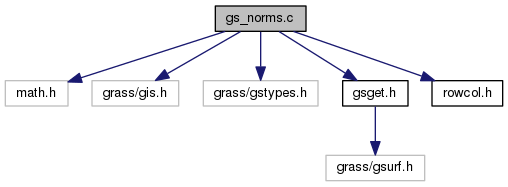GRASS Programmer's Manual  6.5.svn(2014)-r66266
gs_norms.c File Reference

OGSF library - calculation normals (lower level functions) More...

`#include <math.h>`
`#include <grass/gis.h>`
`#include <grass/gstypes.h>`
`#include "gsget.h"`
`#include "rowcol.h"`
Include dependency graph for gs_norms.c:Go to the source code of this file.

## Macros

#define NTOP   0x00001000

#define NBOT   0x00000100

#define NLFT   0x00000010

#define NRGT   0x00000001

#define NALL   0x00001111

#define NTL   0x00001010

#define NTR   0x00001001

#define NBL   0x00000110

#define NBR   0x00000101

#define SET_NORM(i)
This macro is only used in the function calc_norm() More...

## Functions

void init_vars (geosurf *gs)
Init variables. More...

int gs_calc_normals (geosurf *gs)
Calculate normals. More...

int calc_norm (geosurf *gs, int drow, int dcol, unsigned int neighbors)
Calculate normals. More...

## Detailed Description

OGSF library - calculation normals (lower level functions)

GRASS OpenGL gsurf OGSF Library

(C) 1999-2008 by the GRASS Development Team

This program is free software under the GNU General Public License (>=v2). Read the file COPYING that comes with GRASS for details.

Definition in file gs_norms.c.

## Macro Definition Documentation

 #define NALL   0x00001111

Definition at line 32 of file gs_norms.c.

Referenced by gs_calc_normals().

 #define NBL   0x00000110

Definition at line 36 of file gs_norms.c.

Referenced by gs_calc_normals().

 #define NBOT   0x00000100

Definition at line 28 of file gs_norms.c.

Referenced by calc_norm(), and gs_calc_normals().

 #define NBR   0x00000101

Definition at line 37 of file gs_norms.c.

Referenced by gs_calc_normals().

 #define NLFT   0x00000010

Definition at line 29 of file gs_norms.c.

Referenced by calc_norm(), and gs_calc_normals().

 #define NRGT   0x00000001

Definition at line 30 of file gs_norms.c.

Referenced by calc_norm(), and gs_calc_normals().

 #define NTL   0x00001010

Definition at line 34 of file gs_norms.c.

Referenced by gs_calc_normals().

 #define NTOP   0x00001000

Definition at line 27 of file gs_norms.c.

Referenced by calc_norm(), and gs_calc_normals().

 #define NTR   0x00001001

Definition at line 35 of file gs_norms.c.

Referenced by gs_calc_normals().

 #define SET_NORM ( i )
Value:
dz1 = z1 - z2; \
dz2 = z3 - z4; \
temp = (float) -dz1 * y_res_z2; \
temp = (float) dz2 * x_res_z2; \
temp = c_z2; \
normalizer = sqrt(temp * temp + temp * temp + c_z2_sq); \
if (!normalizer) normalizer= 1.0; \
temp[X] /= normalizer; \
temp[Y] /= normalizer; \
temp[Z] /= normalizer; \
PNORM(i,temp);
#define Y(x)
Definition: display/draw.c:246
#define X(y)
Definition: display/draw.c:248
#define PNORM(i, nv)
Definition: gsget.h:56
if(!YY_CURRENT_BUFFER)
Definition: lex.yy.c:799

This macro is only used in the function calc_norm()

Definition at line 42 of file gs_norms.c.

Referenced by calc_norm().

## Function Documentation

 int calc_norm ( geosurf * gs, int drow, int dcol, unsigned int neighbors )

Calculate normals.

Need either four neighbors or two non-linear neighbors passed initial state of neighbors known from array position and data row & col

Parameters
 gs surface (geosurf) drow data row dcol data col neighbors neighbors id
Returns
0 no normals
1 on success

Definition at line 206 of file gs_norms.c.

References BM_get(), DRC2OFF, GET_MAPATT, NBOT, NLFT, NRGT, NTOP, and SET_NORM.

Referenced by gs_calc_normals().

 int gs_calc_normals ( geosurf * gs )

Calculate normals.

OPTIMIZED for constant dy & dx

The norm array is always the same size, but diff resolutions force resampled data points to have their normals recalculated, then only those norms are passed to n3f during drawing. Norms are converted to a packed unsigned int for storage, must be converted back at time of use.

Todo:
fix to correctly calculate norms when mapped to sphere!

Uses the previous and next cells (when available) for normal calculations to produce smoother normals

Parameters
 gs surface (geosurf)
Returns
1 on success
0 on failure

Definition at line 124 of file gs_norms.c.

References calc_norm(), G_debug(), gs_update_curmask(), init_vars(), NALL, NBL, NBOT, NBR, NLFT, NRGT, NTL, NTOP, NTR, VCOLS, and VROWS.

Referenced by GS_get_norm_at_xy(), GS_update_normals(), gsd_coarse_surf_map(), and gsd_surf().

 void init_vars ( geosurf * gs )

Init variables.

for optimization

Parameters
 gs surface (geosurf)

Definition at line 72 of file gs_norms.c.

References gs_get_att_typbuff(), GS_get_scale(), and GS_global_exag().

Referenced by gs_calc_normals().